# Existentially closed

(diff) ← Older revision | Latest revision (diff) | Newer revision → (diff)

existentially complete

Letbe a first-order language (cf. Model (in logic)). A substructureof an-structure(cf. Structure) is called existentially closed (or existentially complete) inif every existential sentence with parameters fromis true in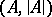if it is true in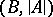. An existential sentence with parameters fromis a closed formula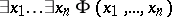, whereis a formula without quantifiers in the first-order language of signature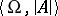, withthe signature of(cf. Model theory).

Ifis a substructure ofandadmits an embedding, fixing the elements of, in some elementary extension of(cf. Elementary theory), thenis existentially closed in. Conversely, ifis existentially closed inandis a cardinal number greater than the cardinality of, thenadmits an embedding, fixing the elements of, in every-saturated extension of(cf. also Model theory).

A memberof a classof-structures is called existentially closed (or existentially complete) with respect toifis existentially closed in every memberof, provided thatis a substructure of.

If a fieldis existentially closed in an extension field, thenis (relatively) algebraically closed in(cf. Algebraically closed field). Hence, a field that is existentially closed with respect to all fields must be algebraically closed, and a formally real field that is existentially closed with respect to all formally real fields must be a real closed field. Existentially closed fields or rings (with respect to suitable classes) give rise to a corresponding Nullstellensatz. This is a theorem describing the form of a polynomialdepending on finitely many other polynomials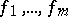, provided that there is an existentially closed memberof the class containing the coefficients of the polynomials and such that every common root of theinis also a root of. For the class of fields, the corresponding theorem is Hilbert's Nullstellensatz (cf. Hilbert theorem). There are corresponding theorems for formally real fields (see Real closed field),-valued fields (see-adically closed field), differential fields, division rings, commutative rings, and commutative regular rings. The general model-theoretic framework was considered by V. Weispfenning in 1977.

How to Cite This Entry:
Existentially closed. Encyclopedia of Mathematics. URL: http://encyclopediaofmath.org/index.php?title=Existentially_closed&oldid=14384
This article was adapted from an original article by F.-V. Kuhlmann (originator), which appeared in Encyclopedia of Mathematics - ISBN 1402006098. See original article